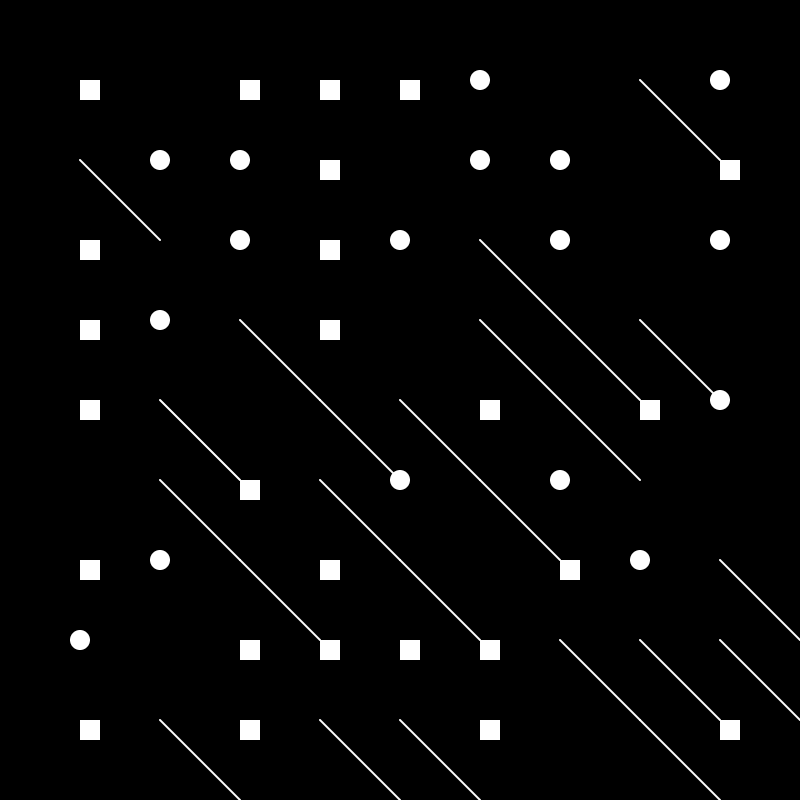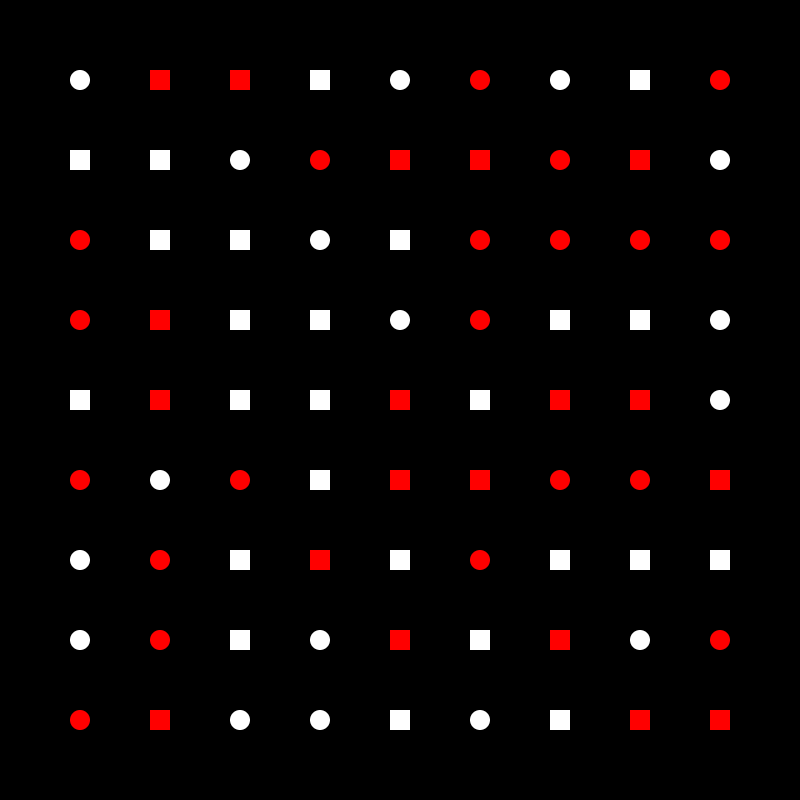# Project: Randomized Pattern

Due Thursday March 4

Create a geometric pattern with elements of randomness combining nested for loops, transformations, conditionals, and the random function.

This is an image-making assignment: don’t make it animated or interactive, a new pattern should be generated upon click.

Follow this tutorial for a template.

Look for inspirations for geometric patterns in art and design.

Check the 10print challenge prompting people to reimagine the famous one line program:

Another use of random: randomize 4 possible shapes (including no shape)```var grid = 40;
function setup() {
createCanvas(400, 400);
background(0);

for (var r = 1; r < width/grid; r++) {
for (var c = 1; c < height/grid; c++) {

var rnd = round(random(0, 3));
print("my random number is "+rnd);

if(rnd == 0) {
noStroke();
ellipse(c * grid, r * grid, 10, 10);
}
if(rnd == 1) {
noStroke();
rect(c * grid, r * grid, 10, 10);
}

if(rnd == 2) {
stroke(255);
line(c * grid, r * grid, c * grid+grid, r * grid+grid);
}
}
}
}
```

### Exercise

Starting from this simple template add randomness to create images like the ones below. Also go through the loop+if exercises if they are not 100% clear.

```var grid = 40;

function setup() {
createCanvas(400, 400);
background(0);
noStroke();

for (var r = 1; r < height/grid; r++) {
for (var c = 1; c < width/grid; c++) {
ellipse(c * grid, r * grid, 10, 10);
}
}
}```